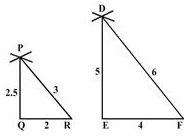Courses

# Test: Construction Of Similar Figures

## 15 Questions MCQ Test Mathematics (Maths) Class 10 | Test: Construction Of Similar Figures

Description
This mock test of Test: Construction Of Similar Figures for Class 10 helps you for every Class 10 entrance exam. This contains 15 Multiple Choice Questions for Class 10 Test: Construction Of Similar Figures (mcq) to study with solutions a complete question bank. The solved questions answers in this Test: Construction Of Similar Figures quiz give you a good mix of easy questions and tough questions. Class 10 students definitely take this Test: Construction Of Similar Figures exercise for a better result in the exam. You can find other Test: Construction Of Similar Figures extra questions, long questions & short questions for Class 10 on EduRev as well by searching above.
QUESTION: 1

### In the figure, DE is parallel to BC such that AE = one fourth of AC. If AB = 6cm, then AD is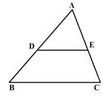​

Solution: Let AC be x. then *(1÷4) + (3÷4)=x AC =1Now In ∆ABC . (by BPL)AD/AB = AE/ACAD=1/4×6AD=1.5 cm
QUESTION: 2

Solution:
QUESTION: 3

### The perimeters of two similar triangles are 25 cm and 15 cm respectively. If one side of first triangle is 9 cm, the corresponding side of the other triangle is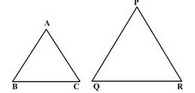​

Solution:

In similar triangles, the perimeter of the triangles will be in the ratio of their corresponding sides.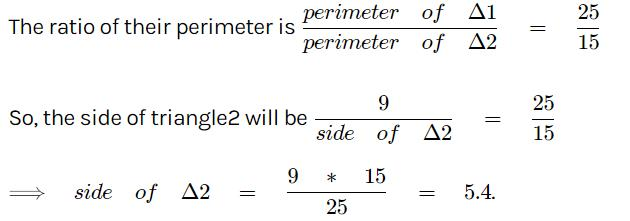QUESTION: 4

In the given figure, AC: CB is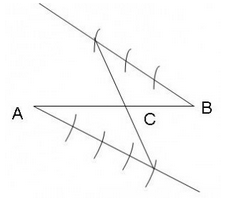Solution:
QUESTION: 5

In the figure QA and PB are perpendiculars on AB. If AO = 10 cm, BO = 6 cm and PB = 9cm, then AQ is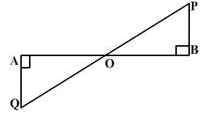Solution:
QUESTION: 6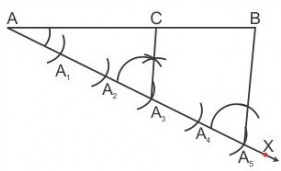From the given figure, find out what is AC : AB.​

Solution:
QUESTION: 7

Sides of two similar triangles are in the ratio 3:8. Areas of these triangles are in the ratio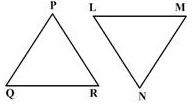Solution:
QUESTION: 8

In the given figure, triangles ABC and DEF are similar. The area of triangle ABC is 9 sq. cm and the area of triangle DEF is 16 sq. cm. If BC = 2.1 cm find the length of EF.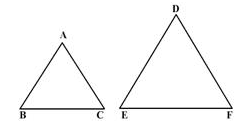Solution:
QUESTION: 9

In the given figure, triangle ABC has been constructed similar to triangle A’BC’. What is the scale factor?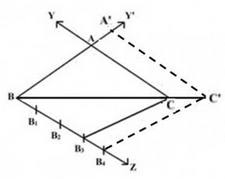Solution:
QUESTION: 10

In the figure, DE is parallel to BC such that AE = one fourth of AC. If AB = 6cm, then AD is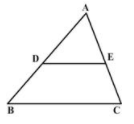Solution:
QUESTION: 11

In drawing triangle ABC, it is given that AB = 3 cm, BC = 2 cm and AC = 6 cm. It is not possible to draw the triangle as:​

Solution:
QUESTION: 12

Identify the incorrect statement.​

Solution:
QUESTION: 13

ABCD is a quadrilateral with BC = 8 cm. Quadrilateral AB’C’D’ is constructed with a scale factor 3/4. What will be the length of B’C’?​

Solution:

The quadrilateral is dilated by a scale factor of 3/4.The shape of image will be same as pre image but size will vary means it will be smaller .If Dilation factor is , means 0< Dilation factor<1, the image is be smaller than preimage.
So new length =k*original size=¾*8 = 6cm
So B’C’ = 6cm

QUESTION: 14

In the given figure, AB and DE are perpendiculars to BC. If AB = 9cm, DE = 3cm and AC = 24cm then AD is equal to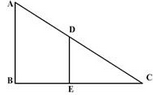Solution:

Since AB||DE so Angle A= Angle D,Angle C is common
So by AA criterion, ΔABC - ΔDEC
So,the corresponding sides are in proportion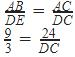DC=8